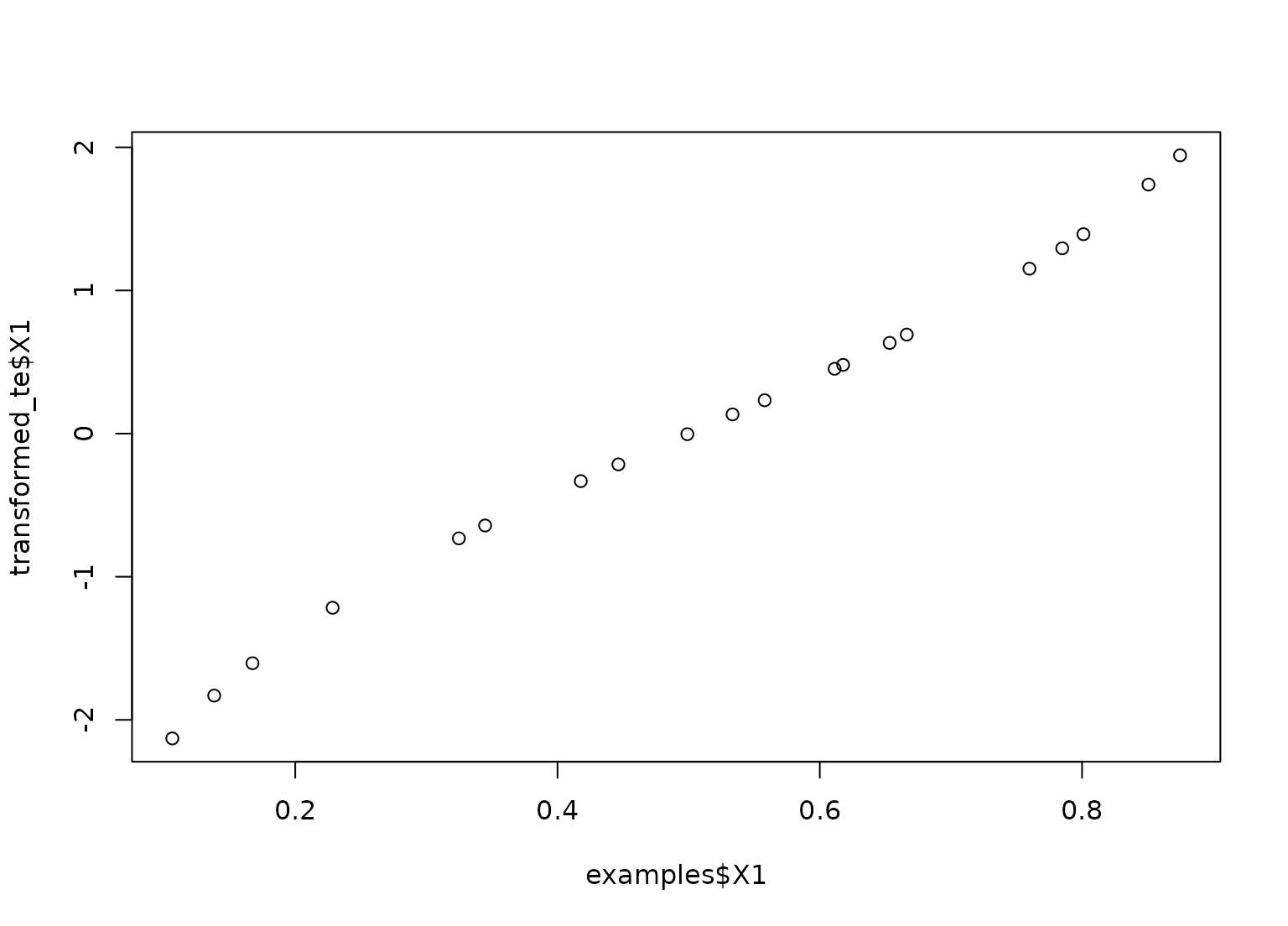step_logit() creates a specification of a recipe step that will logit transform the data.

## Usage

step_logit(
recipe,
...,
offset = 0,
role = NA,
trained = FALSE,
columns = NULL,
skip = FALSE,
id = rand_id("logit")
)

## Arguments

recipe

A recipe object. The step will be added to the sequence of operations for this recipe.

...

One or more selector functions to choose variables for this step. See selections() for more details.

offset

A numeric value to modify values of the columns that are either one or zero. They are modified to be x - offset or offset, respectively.

role

Not used by this step since no new variables are created.

trained

A logical to indicate if the quantities for preprocessing have been estimated.

columns

A character string of the selected variable names. This field is a placeholder and will be populated once prep() is used.

skip

A logical. Should the step be skipped when the recipe is baked by bake()? While all operations are baked when prep() is run, some operations may not be able to be conducted on new data (e.g. processing the outcome variable(s)). Care should be taken when using skip = TRUE as it may affect the computations for subsequent operations.

id

A character string that is unique to this step to identify it.

## Value

An updated version of recipe with the new step added to the sequence of any existing operations.

## Details

The logit transformation takes values between zero and one and translates them to be on the real line using the function f(p) = log(p/(1-p)).

## Tidying

When you tidy() this step, a tibble with columns terms (the columns that will be affected) is returned.

## Case weights

The underlying operation does not allow for case weights.

Other individual transformation steps: step_BoxCox(), step_YeoJohnson(), step_bs(), step_harmonic(), step_hyperbolic(), step_inverse(), step_invlogit(), step_log(), step_mutate(), step_ns(), step_percentile(), step_poly(), step_relu(), step_sqrt()

## Examples

set.seed(313)
examples <- matrix(runif(40), ncol = 2)
examples <- data.frame(examples)

rec <- recipe(~ X1 + X2, data = examples)

logit_trans <- rec %>%
step_logit(all_numeric_predictors())

logit_obj <- prep(logit_trans, training = examples)

transformed_te <- bake(logit_obj, examples)
plot(examples$X1, transformed_te$X1)tidy(logit_trans, number = 1)
#> # A tibble: 1 × 2
#>   terms                    id
#>   <chr>                    <chr>
#> 1 all_numeric_predictors() logit_ooyvr
tidy(logit_obj, number = 1)
#> # A tibble: 2 × 2
#>   terms id
#>   <chr> <chr>
#> 1 X1    logit_ooyvr
#> 2 X2    logit_ooyvr